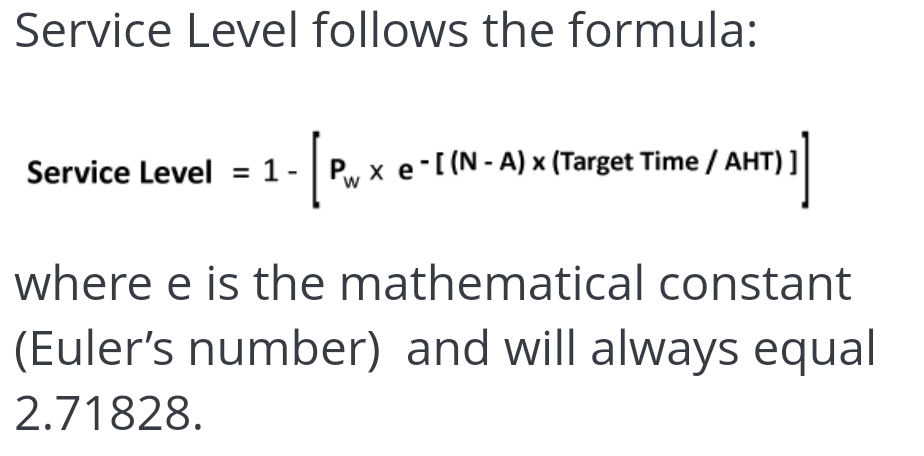top of page
Search
• Vinay Vasudevan

# The Mechanics of Erlang - GoS Model

Updated: Feb 7, 2022

Buck up! It's time for some mathematics.

If you are from WFM, you would have never missed the word "Erlang". This is so famous amongst the WFM folks that it doesn't need any introduction.

Erlang formulas are such a major contributor in the world of WFM

There are many functions we use in Erlang such as Fractional Agents, SLA, AgentsASA, etc.

But what is the workflow behind these formulas? How do they work?

In this article, I'm trying to explain the Erlang formula in a simpler way so that we can understand what is the actual mathematics behind it.

Here are some of the inputs required to calculate FTE and other parameters using Grade of Service (GoS).

• Call Volume per Interval

• AHT

• Interval Length

• Service Goal

• Service Time

Here is the formula from WikipediaPw = Probable Wait Time

A = Raw Agents Required

N = Estimated Agents Required

"A" is pure workload which is Calls*AHT/Interval Length

"N" is A+1, but the +1 is subjected to vary depending upon the additional FTE required to meet SL Goal

I've attached the excel calculations at the end of this article which elaborates the formula with a worked example.

Make sure you check it, without which this article will be incomplete.

Once we calculate the Probable wait time, other parameters can also be calculated using the below formulasIf you closely check the formulas in the sheet, I have rounded up the value of N which is the FTE

This is absurd because we all know that FTE is always in decimal points and not as a whole number

Here, we can't use decimals as there is no option of finding factorials of decimals in mathematics.

We definitely can use the gamma function to find the factorial of decimals, but I'm still in the process of finding the accuracy of it

This calculation opens a lot of new perspectives in WFM which I leave it to users to think about

I would like to end this up with a beautiful quote "A strong economy begins with a strong, well-educated workforce" - Bill Owens

Below is the link for the excel working model I mentioned earlier.

Erlang Working Model - SL

Stay Tuned for the next article!!

Source: Wikipedia and Call Centre Helper